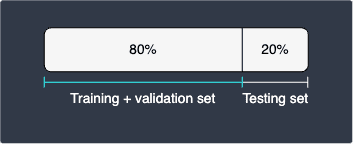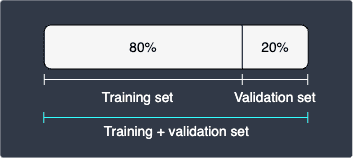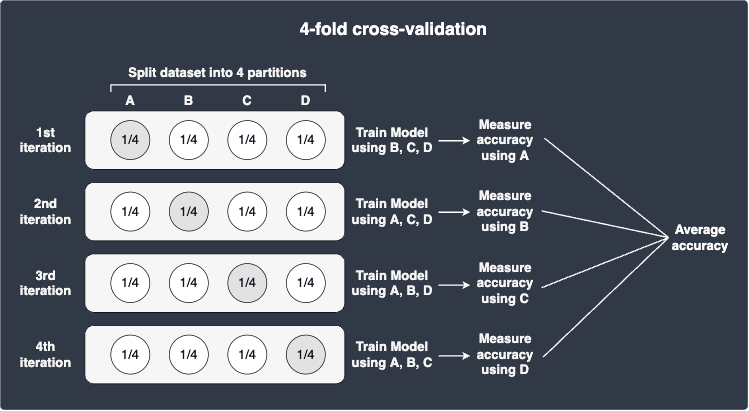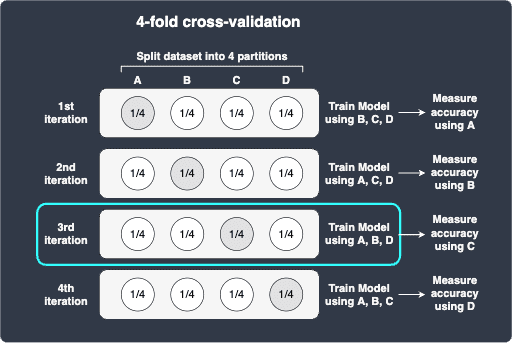search
Search
Map of Data Science
search toc
Thanks for the thanks!
close
account_circle
Profile
exit_to_app
Sign out
search
keyboard_voice
close
Searching Tips
Search for a recipe:
"Creating a table in MySQL"
Search for an API documentation: "@append"
Search for code: "!dataframe"
Apply a tag filter: "#python"
Useful Shortcuts
/ to open search panel
Esc to close search panel
to navigate between search results
d to clear all current filters
Enter to expand content previewDoc SearchCode Search BetaSORRY NOTHING FOUND!
mic
Start speaking...Voice search is only supported in Safari and Chrome.
Shrink
Navigate to
1. ML models
2. Feature engineering
3. Optimization
4. Model evaluation
check_circle
Mark as learned
thumb_up
0
thumb_down
0
chat_bubble_outline
0
auto_stories new
settings

# Comprehensive Guide on Random Search

schedule Mar 5, 2023
Last updated
local_offer
Machine LearningPython
Tags
map
Check out the interactive map of data scienceColab Notebook

You can run all the code snippets in this guide with my Colab Notebook.

lock

# What is random search?

Random search is a technique to determine hyper-parameter values that optimize the performance of machine learning models by random sampling. When building models, we are often in the dark about what values to use for our hyper-parameters. For instance, if we're training a random forest model, we need to choose the desired maximum depth of each tree as well as the number of trees in the forest. Random search allows us to test out a wide range of random combinations to get a general sense of what hyper-parameters perform well.

# Simple example of using random search for hyper-parameter tuning

Suppose we are building a random forest model and we want to know what values to pick for our hyper-parameters. Random forests have the following hyper-parameters:

• `max_depth`: the maximum number of levels of the trees.

• `n_estimators`: the number of trees to train in the forest.

• `max_features`: the maximum number of features to sample when creating each split.

• `bootstrap`: the sampling method, that is, sampling with or without replacement.

Don't worry if you don't know how random forest works and what these hyper-parameters mean - just know that we are dealing with a machine learning model with some hyper-parameters.

## Supply a list of hyper-parameter values to potentially try out

To perform random search, we first need to supply the values of the hyper-parameters to test:

``` max_depth: [3, 5, 7, 10],n_estimators: [10, 100, 1000],max_features: [2, 3, 4],bootstrap: [True, False] ```

Here, we have a total of 72 combinations of hyper-parameter values to potentially try out:

``` 4 * 3 * 3 * 2 = 72 ```

The main idea behind random search is that we do not test all 72 combinations - instead, we randomly select a small number of different combinations to test. In this way, we can test a wide range of combinations to quickly gauge the optimal hyper-parameter values. Grid search, on the other hand, is a brute-force technique that does test all 72 combinations.

## Randomly selecting some combinations of hyper-parameter values for testing

Continuing with this example, suppose we randomly selected the following 4 combinations of hyper-parameter values:

Combination

max_depth

n_estimators

max_features

bootstrap

1

3

10

2

True

2

5

100

2

True

3

7

100

3

False

4

8

1000

4

False

Our goal now is to train our model using these 4 different combinations and determine which combination results in the best performance.

## Splitting dataset into training + validation set and testing set

There are many ways to split our dataset for hyper-parameter tuning and evaluation, but we will only go through one of them here. Let's split the dataset into a pair of training + validation set and testing set like so:The testing set is reserved for measuring the final performance of our model. In the next step, we will further split the training + validation set into a pair of training and validation sets. Note that validation sets are basically testing sets for hyper-parameter tuning, that is, they are for measuring the performance of models trained using different hyper-parameters.

## Computing performance metric for each combination using cross-validation

To keep things simple, suppose we are solving a binary classification problem and the performance metric we want to optimize is classification accuracy.

To compute the accuracy, we typically use a technique called cross-validation. For hyper-parameter tuning, cross-validation further splits the training + validation set above into a pair of training and validation sets:We build the model with a specific combination of hyper-parameter values using the training set, and then compute the performance metric using the validation set. Cross-validation repeats this process multiple times, and each time selects a different pair of training and validation sets. The final output of cross-validation is the average of all the metrics computed.

For instance, suppose we performed 4-fold cross-validation as illustrated below:Suppose cross-validation returned the following accuracies for each combination:

Combination

max_depth

n_estimators

max_features

bootstrap

Accuracy

1

3

10

2

True

80%

2

5

100

2

True

85%

3

7

100

3

False

82%

4

8

1000

4

False

83%

We see that model trained using the hyper-parameters in combination 2 performs the best! We now go back to the models constructed during cross-validation for combination 2. Since we performed 4-fold cross-validation, we have constructed 4 models. Let's assume that 3rd model performed the best, that is, it had the highest validation accuracy:Now that we have the optimal model with the best-performing hyper-parameter values, we want to test how well our model performs for unseen data. We therefore have to refer back to the reserved testing set:We use this testing set to measure the final performance of our model!

WARNING

Strictly speaking, random search does not return the optimal combination of hyper-parameter values. Instead, this technique provides us with a general sense of what ranges of hyper-parameter values work well. To determine the optimal values, we typically perform grid search after random search to further experiment with values around the optimal hyper-parameter values returned by random search.

# Performing random search with scikit-learn

Just like in our simple example, suppose we are building a random forest to solve a classification task. Here is our dataset:

``` import pandas as pddf = pd.read_csv("https://raw.githubusercontent.com/SkyTowner/sample_data/main/iris_data.csv")df.head() sepal_length sepal_width petal_length petal_width species0 5.1 3.5 1.4 0.2 01 4.9 3.0 1.4 0.2 02 4.7 3.2 1.3 0.2 03 4.6 3.1 1.5 0.2 04 5.0 3.6 1.4 0.2 0 ```

The dataset is the classic Iris dataset:

• the first 4 columns are the features.

• the `species` column, which consists of three different categories, is the target.

Our goal is to find a good combination of hyper-parameter values using random search.

Let's start by splitting our dataset into 70% training and 30% testing sets using `train_test_split(~)`:

``` from sklearn.model_selection import train_test_splitdf_features = df.iloc[:,:-1]df_target = df["species"]X_train, X_test, y_train, y_test = train_test_split(df_features, df_target, test_size=0.3, random_state=42) ```

The first step of random search is to select a range of potential hyper-parameter values to try out:

``` param_dist = { "max_depth": [3, 5, 7, 10], "n_estimators": [5, 8, 10], "max_features": [2, 3, 4], "bootstrap": [True, False]} ```

We then initialize our random forest classifier, and feed this into a module called `RandomizedSearchCV`, which returns a model object that we can use to fit our dataset:

``` from sklearn.model_selection import RandomizedSearchCVfrom sklearn.ensemble import RandomForestClassifierrf = RandomForestClassifier(random_state=42)rs_model = RandomizedSearchCV( estimator=rf, param_distributions=param_dist, n_iter=5, cv=4, random_state=42, scoring="accuracy")rs_model.fit(X_train, y_train) ```

Here, note the following:

• `n_iter` is the number of different combinations of hyper-parameter values to randomly test. In this case, we are selecting a total of `5` random combinations. Selecting a high value for `n_iter` means we can test out more combinations, but at the cost of more computing time since each combination requires training and performance tracking via cross-validation.

• `cv` is the number of folds to use for cross-validation - please consult our guide here to learn more.

• `random_state` is for reproducibility. As long as we choose the same value for `random_state`, we will always obtain the same model and evaluation results despite the stochastic nature of random forest.

• `scoring="accuracy"` indicates that the performance metric we want to optimize is accuracy. This means that cross-validation will output the average accuracy based on the testing folds.

• the `fit(~)` method at the end is what performs the random search.

Now that we have performed random search, we can find out the best combination of hyper-parameter values like so:

``` rs_model.best_params_ {'n_estimators': 5, 'max_features': 2, 'max_depth': 3, 'bootstrap': True} ```

With this specific combination of hyper-parameter values, the accuracy of our model is:

``` rs_model.best_score_ 0.9426638176638177 ```

Recall that random search selects different combinations of hyper-parameters randomly. We can look at what combinations were tested using `cv_results_`:

``` rs_model.cv_results_ ...params': [ {'n_estimators': 8, 'max_features': 3, 'max_depth': 3, 'bootstrap': True}, {'n_estimators': 10, 'max_features': 4, 'max_depth': 7, 'bootstrap': False}, {'n_estimators': 5, 'max_features': 2, 'max_depth': 7, 'bootstrap': True}, {'n_estimators': 5, 'max_features': 2, 'max_depth': 3, 'bootstrap': True}, {'n_estimators': 8, 'max_features': 2, 'max_depth': 10, 'bootstrap': True}], 'split0_test_score': array([0.96296296, 0.85185185, 0.92592593, 0.96296296, 0.92592593]), 'split1_test_score': array([0.80769231, 0.84615385, 0.84615385, 0.84615385, 0.84615385]), 'split2_test_score': array([0.96153846, 0.96153846, 0.96153846, 1. , 0.96153846]), 'split3_test_score': array([0.96153846, 0.92307692, 0.96153846, 0.96153846, 0.96153846]), 'mean_test_score': array([0.92343305, 0.89565527, 0.92378917, 0.94266382, 0.92378917]),... ```

Because we set `n_iter=5`, we see that 5 different combinations were tested. The `split0_test_score` is the test scores (accuracies) computed based on the 1st fold of the dataset. This is an array that consists of 5 test scores - one score for each hyper-parameter combination.

We can obtain the best-performing model constructed during random search like so:

``` model_optimal = rs_model.best_estimator_ ```

To compute the accuracy of this model using the reserved testing set:

``` from sklearn.metrics import classification_reporty_test_predicted = model_optimal.predict(X_test)print(classification_report(y_test, y_test_predicted)) precision recall f1-score support 0 1.00 1.00 1.00 19 1 1.00 1.00 1.00 13 2 1.00 1.00 1.00 13 accuracy 1.00 45 macro avg 1.00 1.00 1.00 45weighted avg 1.00 1.00 1.00 45 ```

We see that the testing accuracy is `1.00`, which means that we have a perfect classifier. Don't worry, there's nothing fishy going on here because most classification models have a near-perfect accuracy for the Iris dataset.

In practice, we should go back and experiment with the parameters that we have passed into random search. For instance, we could:

• increase the range of hyper-parameter values to try out.

• set a much higher value for `n_iter` so that more combinations can be sampled in search of better hyper-parameter values. This is especially important if we have a wide range of hyper-parameter values that we wish to try out.

• experiment with a different number of folds (`cv`). Consult our cross-validation guide to learn more about folds.

# Final remarks

If we wish to experiment with a large number of hyper-parameters of models that take a long time to train, then we should opt for random search instead of grid search. This is because random search allows us to efficiently understand the rough range of hyper-parameter values that work well. Afterwards, we can use grid search to fine-tune the hyper-parameters to improve the performance even more!

thumb_up
thumb_down
Comment
Citation
Ask a question or leave a feedback...
thumb_up
0
thumb_down
0
chat_bubble_outline
0
settings
Enjoy our search
Hit / to insta-search docs and recipes!